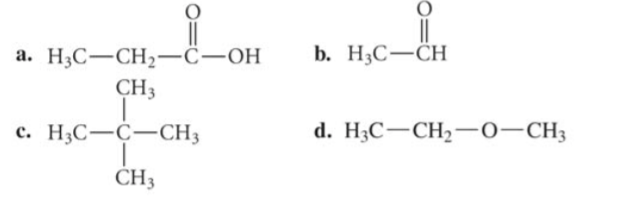# Problem: Identify the family to which the compound (a) belongs.

###### FREE Expert Solution

Recall the following functional groups:

• Alkane → hydrocarbons with C-C single bond only
• Alkene → hydrocarbons with C-C double bond/s
• Alkyne → hydrocarbons with C-C triple bond/s
• Alcohol → R-OH; where R is an alkyl group
• Aldehyde → R-CHO; where R is an alkyl group
• Ether → R-O-R' → where R and R' are alkyl groups
• Ester → RCOOR' → where R and R' are alkyl groups
• Ketone → RC(=O)R'; where R and R' are alkyl groups
• Amine → R-NH2; where R is an alkyl group
• Amide → RC(=O)NR′R″; where R, R′, and R″ represent organic groups or hydrogen atoms
• Carboxylic acid → R-COOH; where R is an alkyl group
95% (257 ratings)###### Problem Details

Identify the family to which the compound (a) belongs.Frequently Asked Questions

What scientific concept do you need to know in order to solve this problem?

Our tutors have indicated that to solve this problem you will need to apply the Functional Groups in Chemistry concept. You can view video lessons to learn Functional Groups in Chemistry. Or if you need more Functional Groups in Chemistry practice, you can also practice Functional Groups in Chemistry practice problems.